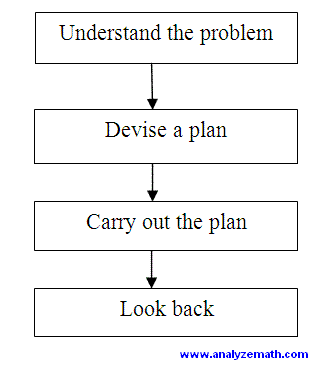# Skills Needed for Mathematical Problem Solving (2)

2. MATHEMATICAL PROBLEM SOLVING AS A PROCESS

Mathematical problem solving is a process depending on many phases. There are four main phases in mathematical problem solving .

1. Understand the problem
2. Devise a plan
3. Carry out the plan
4. Look back

 2. MATHEMATICAL PROBLEM SOLVING AS A PROCESS Mathematical problem solving is a process depending on many phases. There are four main phases in mathematical problem solving . Understand the problem Devise a plan Carry out the plan Look back In solving a problem similar, or close, to another problem solved in the past, we will surely follow a linear process as shown in figure 1. The term linear is used to mean that the learner goes from one phase to another until the problem is solved.Fig. 1: Four steps in mathematical problem solving. (linear model) However this linear process may not be enough when solving a genuine and challenging problem . It is a possible that we carry out the plan but it does not give the right answer or it does not even work. We therefore need to look back critically at the formulation of the problem as well as the information given in the problem. A more practical model of the process is shown in figure 2. The learner need to understand that in solving a problem he needs to look back and reexamine the whole problem during the problem solving process and be ready to restart again if necessary.Fig. 2: Four steps and looking back at the problem. (More practical model) One of the difficulties in problem solving is the fact that several steps are needed to solve a given problem. Also at each step, students need to use several skills. There are also many factors and skills that determine the outcome of the process. 1, 2, 3, 4, 5, 6, 7,